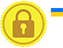# Conditions in javascript?

Conditions are used to check a specific criteria.

It will return either true or false value.

Condition Operators
Symbol Description
`==` Equal
`<` Less than
`>` Greater than
`<=` Less than or equal
`>=` Greater than or equal
`!=` Not equal
`===` equal with value and type
Condition Explanation
Condition Description
`a == b` Return true, if a and b values are same.
`a <= b` Return true, if a value is smaller than b value or a and b values are same.
`a >= b` Return true, if a value is bigger than b value or a and b values are same.
`a != b` Return true, if a and b values are not same.
`a === b` Returns true, if a and b values and types are same.
Condition Examples
Condition Description
`10 == 20` False
`10 <= 20` False
`10 >= 20` True
`10 != 20` True
`10 === 20` False

# Difference between == and ===

Condition Result
`10 == 10` True
`10 == "10"` True
`10===10` True
`10 === "10"` False

# Condition Workout CodePlay

Below some condition are given for you to workout, check the conditions and select either true or false.

x=40

y=100

z=40

`x > y`
`z > x`
`z <= x`
`z >= x`
`y == z`
`y > z`
`x < y`
`x === z`